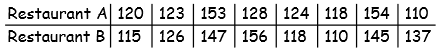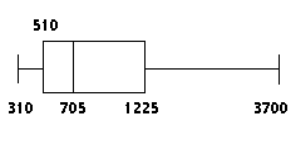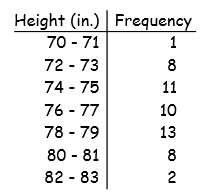# Иностранная литература и современность: General Statistics: Ch 3 Test Flashcards

Set Details Share
created 9 years ago by GreenHero64
11,701 views
This course is designed to acquaint the student with the principles of descriptive and inferential statistics. Topics will include: types of data, frequency distributions and histograms, measures of central tendency, measures of variation, probability, probability distributions including binomial, normal probability and student's t distributions, standard scores, confidence intervals, hypothesis testing, correlation, and linear regression analysis. This course is open to any student interested in general statistics and it will include applications pertaining to students majoring in athletic training, pre-nursing and business.
College: First year, College: Second year, College: Third year, College: Fourth year
Subjects:
statistics, mathematics, probability & statistics
Page to share:
Embed this setcancel
COPY
code changes based on your size selection
Size:
X

1

Suppose that all the values in a data set are converted to z-scores. Which of the statements below istrue?

The mean of the z-scores will be 0, and the standard deviation of the z-scores will be 1.

2

Find all measures of center: A store manager kept track of the number of newspapers sold each week over a randomly selected seven-week period. The results are shown below.

80 39 214 152 264 239 232

Find the median number of newspapers sold.

Mean: = 174.3 newspapers

Median: x(~) = 214.0 newspapers

Midrange: 151.5

Mode: none

3

Which is better:

A score of 82 on a test with a mean of 70 and a standard deviation of 8, or a score of 82 on a test with a mean of 75 and a standard deviation of 4?

The second 82

(82 – 70) ÷ 8 (82 – 75) ÷ 4

= 1.5 = 1.75

4

A department store, on average, has daily sales of \$29,876.76. The standard deviation of sales is \$1000.On Tuesday, the store sold \$34,893.71 worth of goods.

Find Tuesday's z score.

Was Tuesday an unusually good day?

5.02, yes

(34,893.71 – 29,876.76) ÷ 1000

= 5.01695

5Find the range, variance, and standard deviation for each of the two samples, then compare the two sets of results: When investigating times required for drive-through service, the following results (in seconds) were obtained.

Restaurant A: 44 sec; s2 = 260.8 sec2; s = 16.1 sec

Restaurant B: 46 sec; s2 = 285.6 sec2; s = 16.9 sec

There is more variation in the times for restaurant B.

6

Find the z-score corresponding to the given value and use the z-score to determine whether the value is unusual.

A time for the 100 meter sprint of 21.3 seconds at a school where the mean time for the 100 meter sprint is 17.5 seconds and the standard deviation is 2.1 seconds.

1.80; not unusual

(21.3 – 17.5) ÷ 2.1

= 1.80952381

7

Use the given sample data to find Q3:

49 52 52 52 74 67 55 55

61.0

8

The weekly salaries (in dollars) of 24 randomly selected employees of a company are shown below.

Construct a boxplot for the data set.

310 320 450 460 470 500 520 540

580 600 650 700 710 840 870 900

1000 1200 1250 1300 1400 1720 2500 3700The​ 5-number summary is 310​, 510​, 705​, 1225, 3700

9The heights of a group of professional basketball players are summarized in the frequency distribution below.

Find the mean height.

x̄ = 76.7 in.

(Use calculator)

L1 L2

midpt freq

1–Var Stats L1,L2

mean = 76.68867925

10

The systolic blood pressure of 18-year-old women is normally distributed with a mean of 120 mmHg and a standard deviation of 12 mmHg. What percentage of 18-year-old women have a systolic blood pressure between 96 mmHg and 144 mmHg?

95%

(96 – 120) ÷ 12 (144 – 120) ÷ 12

= -2SD = 2SD

2SD = 95%